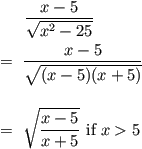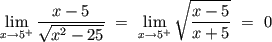1. We are reviewing limits again and Im lost on this problem. Could someone walk me through it...

(x-5)(x^(2)-25)^(-.5)

When I find the derivative of the bottom am I supposed to look at it as if it were to the negative one half or positive one half? That is really my main confusion.2.

3. Just to clarify... is the function (x-5)/sqrt(x<sup>2</sup>-25)? And if this is a limit problem as in
"lim x-->something of something," it would help to see the actual limit portion of it...4. Yes you are correct. And it is the limit as x approaches 55. Ok, so lim x-->5 (x-5)/sqrt(x<sup>2</sup>-25) equals, as I'm sure you've realized, 0/0, which is an indeterminate form. So, as you seem to realize, you need to use L'Hopital's rule from here. When you use L'Hopital's rule, you take the derivatives of the top and bottom separately (so you won't be using the quotient rule). With that, I think you should have an answer to your question about the square root...6. The important point that Chemboy makes is that, to apply l'Hopital's rule, you need to be looking at a ratio of functions. You need a function on top and a function on bottom. The original way you wrote this did not display this fact, so on the surface it wouldn't even make sense to apply l'Hopital's rule.7. So then would I write the first derivative as 1/1/4xsqrt(x2-25) which would just be 4xsqrt(x2-25)?8. Check that bottom derivative again.9. You do not need L’Hôpital’s rule here. Observe thatHenceNote that the limit has to approach from the right as the function is not defined for −5 < x < 5.10.Originally Posted by JaneBennet
You do not need L’Hôpital’s rule here. Observe thatHenceNote that the limit has to approach from the right as the function is not defined for −5 < x < 5.
JaneBennet, how do you write that in your post? (the sqrt and division)11. Well, I formatted the equations using LaTeX on a math site that has LaTeX installed, then I saved and uploaded the GIF images.There are plenty of math sites on which you can type equations in LaTeX – http://www.mathisfunforum.com/index.php was where I did the above samples.12. Ok, thanks :-DBookmarks
 Posting Permissions
 You may not post new threads You may not post replies You may not post attachments You may not edit your posts   BB code is On Smilies are On [IMG] code is On [VIDEO] code is On HTML code is Off Trackbacks are Off Pingbacks are Off Refbacks are On Terms of Use Agreement